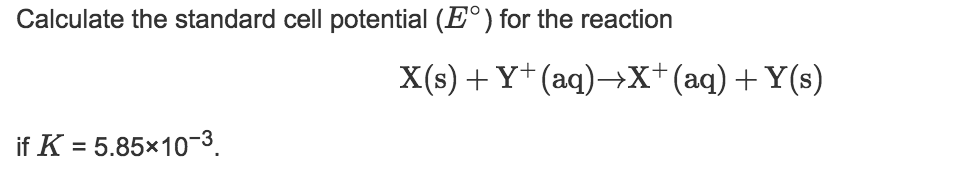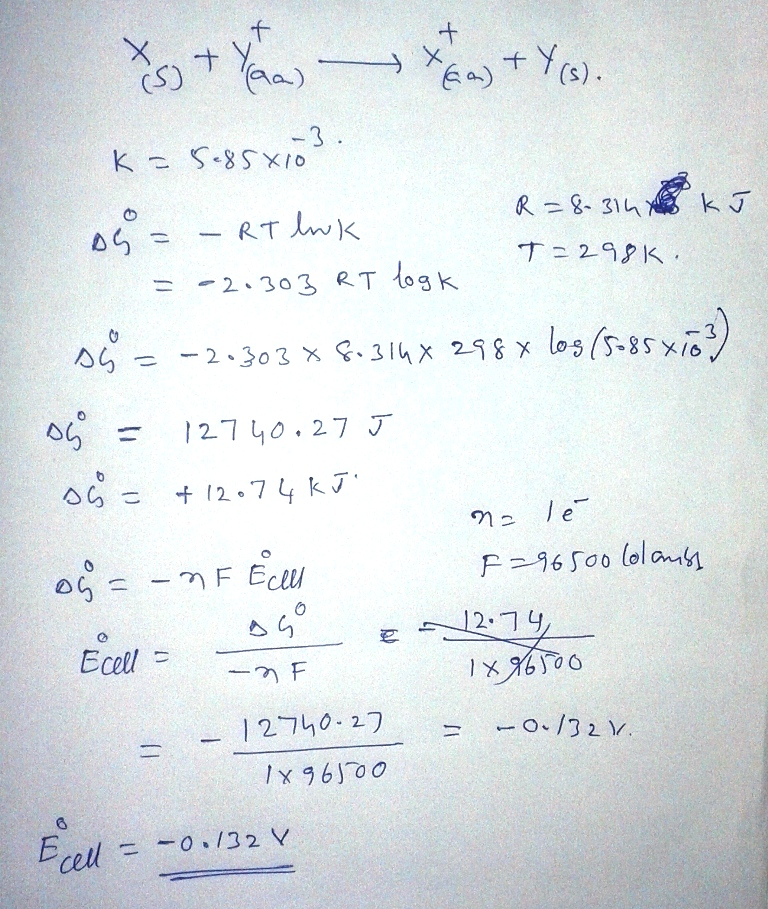# Question & Answer: Calculate the standard cell potential (E degree) for the reaction X(s) + Y^+(aq) rightarrow X^…..Calculate the standard cell potential (E degree) for the reaction X(s) + Y^+(aq) rightarrow X^+(aq) + Y(s) if K = 5.85 times 10^-3.